Function Repository Resource:

# FindDistanceInstance

Find an instance of n-dimensional vectors that produce a specified distance matrix

Contributed by: Rob Knapp, Paritosh Mokhasi
 ResourceFunction["FindDistanceInstance"][mat,n] finds an instance of n-dimensional vectors that will generate the specified distance matrix mat.

## Details and Options

mat must be a symmetric square numeric matrix.
n must be a positive integer.
ResourceFunction["FindDistanceInstance"] takes the option and returns a result if the vectors have the specified distances within the specified tolerance t. The default Tolerance value is 10-8.

## Examples

### Basic Examples (2)

Find a set of three 2D points that are equidistant:

 In:=Out=Verify that the points produce the specified distance matrix:

 In:=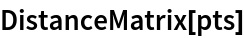Out=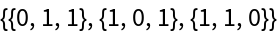### Scope (3)

Find a set of four 3D points that are equidistant:

 In:=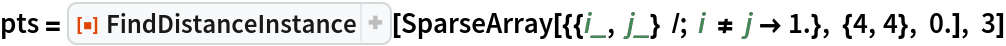Out=Verify that the points are equidistant:

 In:=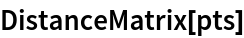Out=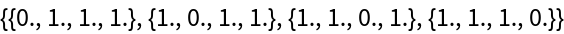The points form a regular tetrahedron in 3D:

 In:=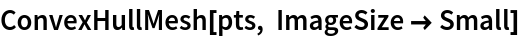Out=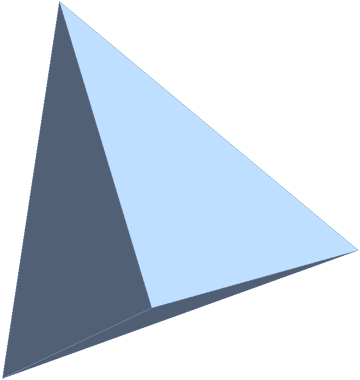### Options (2)

#### Tolerance (2)

A 4D Hilbert matrix with its diagonal zeroed cannot be a distance matrix because it fails to satisfy some triangle inequalities:

 In:=Out=Use a larger tolerance to get a result anyway:

 In:=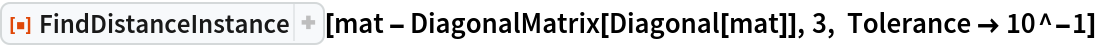Out=### Applications (2)

Find a set of three 2D points whose distances form a HilbertMatrix:

 In:=Out=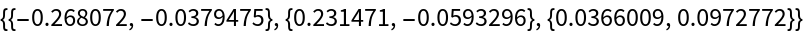In:=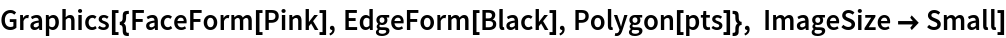Out=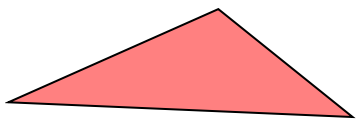Find a set of three 2D points whose distances form a Toeplitz matrix:

 In:=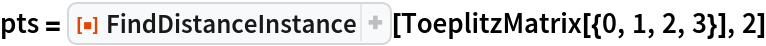Out=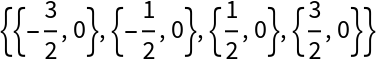In:=Out=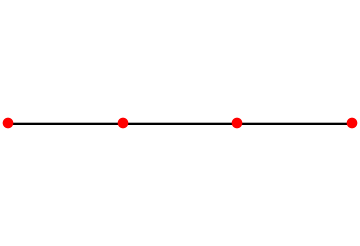Paritosh Mokhasi

## Version History

• 1.0.0 – 10 April 2020